# GMAT Math : DSQ: Calculating the volume of a cube

## Example Questions

### Example Question #1 : Dsq: Calculating The Volume Of A Cube

Find the volume of the cube.

1. The cube has a diagonal of 17.32 inches.

2. The cube has a surface area of 600 square inches.

Statement 2 alone is sufficient, but statement 1 alone is not sufficient to answer the question.

statement 1 alone is sufficient, but statement 2 alone is not sufficient to answer the question.

Each statement alone is sufficient.

Statements 1 and 2 together are not sufficient.

Both statements taken together are sufficient to answer the question, but neither statement alone is sufficient.

Each statement alone is sufficient.

Explanation:

To find the volume of a cube, we only need the length of one side. Using statement 1, we can figure out the length of a side based on the diagonal. We can use the figure below to find the ratio of the diagonal to one side. If the we let the length of one side be x, we can use Pythagorean's theorem to find the length of the diagonal. So, in triangle BCD, we have a right triangle, with two sides of length x. We can set up the equation that the length of BD is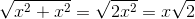.

Then we can see triangle ADB is also a right triangle. Using Pythagorean's theorem we get the length of AB is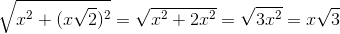.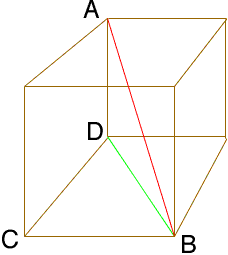So, if we divide the number from statement 1 by the square root of 3, we get the length of each side of the cube. Doing this, we get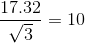. Thus, we can solve this problem with just the information from statement 1.

Now, we can also check statement 2. If we know the surface area of the cube, we can use that information to find the length of each side of the cube. We know that the surface area of a cube is the sum of the six faces of the cube, which all have equal area and are all squares. We can divide the total surface area by 6 to find the surface area of each square face. So, 600/6 = 100. We know that the area of a square is just the length of one side squared, so we can take the square root of 100 to find that the length of each side is 10. Thus statement 2 is also sufficient to solve this problem.

Therefore, the answer is that either statement alone is sufficient to answer the question.

### Example Question #2 : Dsq: Calculating The Volume Of A Cube

How much does a cube weigh?

Statement 1: The cube is made of material that weighs 3 pounds per cubic foot.

Statement 2: Each face of the cube is a square with area 16 square feet.

EITHER statement ALONE is sufficient to answer the question.

Statement 1 ALONE is sufficient to answer the question, but Statement 2 ALONE is NOT sufficient to answer the question.

BOTH statements TOGETHER are sufficient to answer the question, but NEITHER statement ALONE is sufficient to answer the question.

BOTH statements TOGETHER are insufficient to answer the question.

Statement 2 ALONE is sufficient to answer the question, but Statement 1 ALONE is NOT sufficient to answer the question.

BOTH statements TOGETHER are sufficient to answer the question, but NEITHER statement ALONE is sufficient to answer the question.

Explanation:

The weight of a cube is dependent on its density in pounds per cubic foot and its volume in cubic feet. We need Statement 1 for the density. Statement 2 is needed for the volume - and it gives us the means to find it, since we can take the square root of the area of one side, 16, to get sidelength 4 feet, and we can cube that to get the volume of 64 cubic feet. Now we can multiply 64 cubic feet by 3 pounds per cubic foot to get 192 pounds.

### Example Question #3 : Dsq: Calculating The Volume Of A Cube

Find the volume of cube W.

I) The base of W has an area ofleagues squared.

II) The diagonal of the base of W has a length of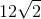leagues.

Both statements taken together are sufficient to solve the question.

Statement 2 is sufficient to solve the question, but statement 1 is not sufficient to solve the question.

Neither statement is sufficient to solve the question. More information is needed.

Statement 1 is sufficient to solve the question, but statement 2 is not sufficient to solve the question.

Each statement alone is enough to solve the question.

Each statement alone is enough to solve the question.

Explanation:

If we know the base of W, we can find the side length. We then cube the side length to find the volume of a square.

If we know the diagonal of the base of W, we can find the side length. As outlined above, we can then cube the side length to find the volume.

Therefore either statement alone is sufficient to solve the question.

### Example Question #4 : Dsq: Calculating The Volume Of A Cube

Calculate the volume of a cube.

1. The length of the cube's edge is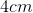.
2. The surface area of the cube is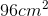.

Statements 1 and 2 are not sufficient, and additional data is needed to answer the question.

Each statement alone is sufficient to answer the question.

Statement 1 alone is sufficient, but statement 2 alone is not sufficient to answer the question.

Both statements taken together are sufficient to answer the question, but neither statement alone is sufficient.

Statement 2 alone is sufficient, but statement 1 alone is not sufficient to answer the question.

Each statement alone is sufficient to answer the question.

Explanation:

Statement 1: We need the length of the edge of the cube to calculate the volume. In this statement we're provided with the length so we just need to plug it into the equation for a cube's volume.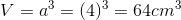Statement 2: In this case, we need to solve for the length of the cube's edge which we can easily do: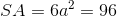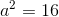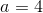Now that we have our length, we can calculate the volume.### Example Question #5 : Dsq: Calculating The Volume Of A Cube

A lab has designed a cube to help with their testing procedures. Find the volume of the cube.

I) The cube will have a side length ofmeters.

II) The cube will have a diagonal of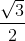meters.

Both statements are needed to answer the question.

Statement I is sufficient to answer the question, but statement II is not sufficient to answer the question.

Either statement is sufficient to answer the question.

Statement II is sufficient to answer the question, but statement I is not sufficient to answer the question.

Either statement is sufficient to answer the question.

Explanation:

Recall that the volume of a cube is equal to the cube of its side length, and that the diagonal of a cube is equal to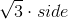length.

I) Use the following: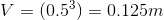II) Gives us the diagonal. Divide by the square root of three, then cube it!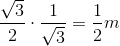Either statement is sufficient to answer the question.

Tired of practice problems?

Try live online GMAT prep today.